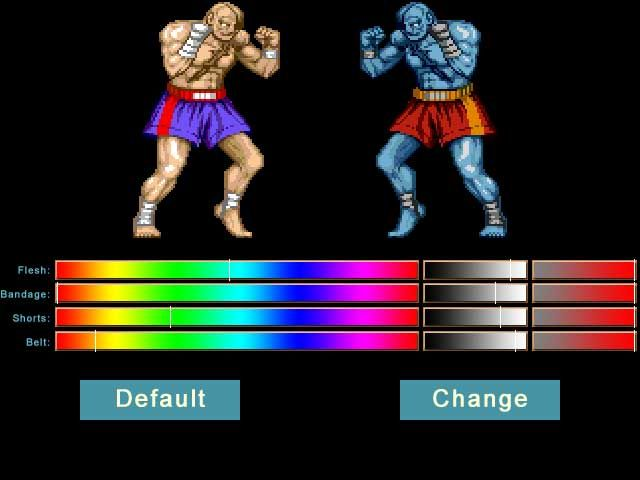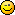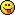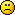## News:

*NEW* Current Version on STEAM16.026
Logfile: EN, DE

Webchat:
Visit the chat

## Change a sprite's hue, saturation and brightness

#### Wampus

UPDATE!

The function ShiftColorValue has been improved. The calculations have been optimised and it only does the minimum  work needed to get its result. You can now also saturate and brighten the original sprite more than the original values. All round a better approach. The new routine has been attached to this post.

See my second post below on this thread for an explanation of the ShiftColorValue function

Back to OP:-

---------------------------

Wrote a routine to see how I could use SPRITE2MEM and MEM2SPRITE to create new sprites with different colour schemes. This sort of thing was seen a lot in the 16-bit era. Example screenshot:-In this demo you can adjust the hue (rainbow bar), the brightness (dark to light bar) and saturation (gray to red bar) of Sagat's flesh, bandages, belt and shorts. Click 'Change' to have a look at the new sprite you've created with your settings and 'Default' to return to defaultJust compile HueShiftExample.gbap to have a look.

#### Kitty Hello

#1
Wow, that's handy!

#### Albert

#2
WoW!
Exactly what I need.

#### Moru

#3
Nice, just what we were discussing last year :-)

#### Hark0

#4
Cool!http://litiopixel.blogspot.com
litiopixel.blogspot.com - Desarrollo videojuegos Indie · Pixel-Art · Retroinformática · Electrónica Development Indie Videogames · Pixel-Art · Retrocomputing · Electronic

#### Wampus

#5
Ok, so clear explanation of ShiftColorValue function

newcolor% = ShiftColorValue ( oldcolor%,  brightness%,  saturation%,  hue% )

The variable newcolor is given a value in RGBA calculated from oldcolor according to settings brightness, saturation and hue.

brightness can be given values from 0 to 200. 0 is totally black. 200 is totally white. 100 is normal brightness.

saturation can be given values from 0 to 200. 0 is totally colourless - black & white. 200 is maximum saturation. 100 is normal saturation. Be careful using values above 100. While this can work well much of the time it can produce odd results in some sprites when brightness is over 100.

hue can be given values from 0 to 359. 0 is no change whatsoever. 180 results in the complete opposite colour.

Code (glbasic) Select
`FUNCTION ShiftColorValue: color, bri, sat, hue// color is the original color to change according to the following values:-// bri = brightness. Can be 0 to 100 to 200. 0 = total darkness. 100 = normal light. over 100 you'll get a bleached look - "over" brightness// sat = saturation. Can be 0 to 100 to 200. 0 = black & white. 100 = normal saturation. over 100 is "over" saturation. More colourful.// hue = hue shift. Can be 0 to 359. 0 is no change.LOCAL hu, cmax%, cmin%, diff, r%, g%, b%, a%, tmin%, tdiff%a = bAND(ASR(color,24),0xff)IF a = 0 RETURN color ELSE r = bAND(color,0xff) g = bAND(ASR(color,8),0xff) b = bAND(ASR(color,16),0xff) cmax = MAX(b,MAX(r,g)) cmin = MIN(b,MIN(r,g)) diff = cmax-cmin // hue calculations - hu = hue of color% IF hue <> 0 IF r > g IF g > b // 7 Between red and yellow hu = ((g-b)/diff)*60 ELSEIF b > g IF b = r hu = 300 // Pure Magnenta ELSE hu = 300 + ((r-b)/diff)*60 // 12 Between Magnenta and Red ENDIF ELSE // g = b hu = 0 // 1 Pure Red ENDIF ELSEIF g > b IF b > r // 9 Between green and cyan hu = 120 + ((b-r)/diff)*60 ELSEIF r > b // 8 Between yellow and green IF r = g // Pure Yellow hu = 60 ELSE hu = 60 + ((g-r)/diff)*60 // 8 Between yellow and green ENDIF ELSE // b = r hu = 120 // 3 Pure green ENDIF ELSE // b > r IF r > g // 11 Between blue and magnenta hu = 240 + ((r-g)/diff)*60 ELSEIF g > r IF g = b // Pure Cyan hu = 180 ELSE hu = 180 + ((b-g)/diff)*60 // 10 Between cyan and blue ENDIF ELSE // r = g hu = hu = 240 // 5 Pure blue ENDIF ENDIF // Adjust hue hue = hue + hu IF hue >= 360 THEN DEC hue, 360 IF hue > 0 AND hue < 60 // Between red and yellow r = cmax b = cmin g = cmin+(hue*(diff/60)) ELSEIF hue > 60 AND hue < 120 // Between Yellow and green g = cmax b = cmin r = cmax-((hue-60)*(diff/60)) ELSEIF hue > 120 AND hue < 180 // Between green and cyan g = cmax r = cmin b = cmin+((hue-120)*(diff/60)) ELSEIF hue > 180 AND hue < 240 // Between cyan and blue b = cmax r = cmin g = cmax-((hue-180)*(diff/60)) ELSEIF hue > 240 AND hue < 300 // Betweeen blue and magnenta b = cmax g = cmin r = cmin+((hue-240)*(diff/60)) ELSEIF hue > 300 // Between mangenta and red r = cmax g = cmin b = cmax-((hue-300)*(diff/60)) ELSEIF hue = 0 // Pure Red r = cmax g = cmin b = cmin ELSEIF hue = 60 // Pure Yellow r = cmax g = cmax b = cmin ELSEIF hue = 120 // Pure Green r = cmin g = cmax b = cmin ELSEIF hue = 180 // Pure Cyan r = cmin g = cmax b = cmax ELSEIF hue = 240 // Pure Blue r = cmin g = cmin b = cmax ELSEIF hue = 300 // Pure magnenta r = cmax g = cmin b = cmax ENDIF ENDIF // Adjust saturation IF sat <> 100 sat = 100-sat IF r <> cmax tmin = r tdiff = cmax - tmin r = tmin + tdiff*(sat/100) ENDIF IF g <> cmax tmin = g tdiff = cmax - tmin g = tmin + tdiff*(sat/100) ENDIF IF b <> cmax tmin = b tdiff = cmax - tmin b = tmin + tdiff*(sat/100) ENDIF ENDIF // Adjust brightness IF bri <> 100 IF bri = 0 r = 0 g = 0 b = 0 ELSE DEC r, (100-bri)*2.55 DEC g, (100-bri)*2.55 DEC b, (100-bri)*2.55 ENDIF ENDIF IF r < 0 THEN r = 0 IF r > 255 THEN r = 255 IF g < 0 THEN g = 0 IF g > 255 THEN g = 255 IF b < 0 THEN b = 0 IF b > 255 THEN b = 255 RETURN bOR(RGB(r,g,b), ASL(a, 24)) ENDIFENDFUNCTION`

#### Quentin

#6
Cool thingI must admit, I've never used MEM2SPRITE until now. Is it fast enough to be used for ingame sprite manipulation? Or should it be used with care?

#### Marmor

#7
its a nice one .  thx

#### Wampus

#8
Quote from: Quentin on 2011-Jan-29
Cool thingI must admit, I've never used MEM2SPRITE until now. Is it fast enough to be used for ingame sprite manipulation? Or should it be used with care?

IMO not a good idea to use it on the fly each frame, i.e. in-game. Even if you increased speed by greatly reducing the number of colours you have to work with by keeping them in a pre-calculated list and skipped alpha channel with 0 value it would still be slow even on relatively fast PCs. Its quick, but not that quick.

The kind of practical thing you can do is re-colour a whole set of sprites on a sprite sheet for use later. In this way its not unlike loading a whole new set of sprites (and about as quick). When actually in use they'd work just the same as the original sprites. For example, in a real-time strategy game you could allow the user to make personalised colour changes to their standard set of units before a game starts. Same with a fighting game like the classic 2D versions of Street Fighter games. Another example would be a character creation screen for an RPG. You could allow the user to customise the colour scheme for their player characters in addition to all the other settings.

#### r0ber7

#9
Hey Wampus,

Check this out.Muchas gracias!

Note: low framerate is due to Fraps, game runs smoothly.#### Wampus

#10
Very niceIncidentally, I'm going to use hue shifting amongst other things to make the appearance of wizards in my Chaos remake easy to change too.

#### Qedo

#11
Can anyone of good will submit the HueShiftExample.gbap project?#### Qedo

#12
Quote
Can anyone of good will submit the HueShiftExample.gbap project?nobody?

#### Kitty Hello

#13
I think when uploading the stuff to the new server, the newlines of the zip and png files were changed. But I don't have the original filey anymore. I'll try to find an old backup, but it doesn't look good#### Qedo

#14
thanks Gernot I understand that there are server backup problems but I also trust in the contribution of the users.
If each of us collaborates, by sending the attachments, we can (slowly) repopulate the forum